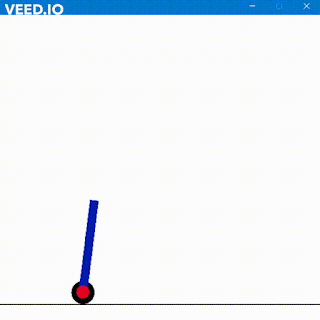### I2C Verilog Code Explanation I

In this post, I am going to explain my previous post regarding I2C. You can visit the post by clicking here.

INOUT  SDA: The SDA line is the inout port because Master will send data, address along this line as well as the Slave will send ACK/ NACK along the same SDA line hence it has to be inout type.

OUTPUT REG SCL: The SCL line will be the output from Master to other Slaves. SCL is controlled by Master here by the register "a" in the code.

REG DIRECTION: This register will decide whether the direction of flow of data on the SDA line. The line assign sda = direction?alpha:1'bz. using the direction keyword.

Its equivalent code will be
if(direction==1)
sda = alpha;
else if(direction==0)
sda = 1'bz;

If Master sets the direction as 1 then sda = alpha. At the same moment, Slave must also have the direction set to 0 in order to allow data from Master. When the Slave wants to send the data then the Slave will set the direction as 1 and Master will set it as 0.

REG ALPHA: This register holds the bit that has to be sent on the SDA line. Since SDA is a wire, therefore it is not possible to use it inside always@ block.

REG [6:0] ADDRESS: This 7-bit register holds the 7bit register holds the address of the slave.

REG[7:0] TEMP: This 8-bit register holds the address of the slave along with RW bit that has to be sent on the SDA line.

REG[7:0] TEMP_RESERVED: This 8-bit register just holds a copy of TEMP register for future use.

TEMP = {ADDRESS,RW}: This concatenates the 7-bit address and 1 bit RW into an 8-bit register and stores the final data back in TEMP.
always @(posedge clk)begin
if(a==0)
scl <= 1;
else if(a==1)
scl <= !scl;
end

Here "a" is a switch. When "ON" it will clock the SCL line. When OFF it will pull up the SCL line HIGH. It is just a manual control for Master to control SCL.

always @(negedge sda)
a <= 1;
As soon as the SDA line is pulled down, "a" is triggered to start SCL clock. This is the START condition. Although SDA has many negative edges it won't affect SCL because of above code. When we want a STOP condition then SDA is pulled HIGH first. Thus no negative edge occurs after that point and then "a" is triggered low which pulls SCL HIGH and STOP condition is achieved.
always @(negedge scl)begin
#1 forever #2 scl2 <= !scl2;
SCL2 is an internal clock which starts as soon as SCL is triggered. This is done because we cannot use SCL for our operation. SDA only changes when SCL is LOW (See the LAST image in my post) and not on positive edge or negative edge. #1 is the delay of 1ns. SCL2 starts ticking after 1ns start of SCL. Using the posedge of SCL2 we achieve our required condition to change SDA when SCL is LOW.

Look at this image above. SDA changes when SCL is low (in middle). It neither changes at positive edge nor at the negative edge.

SDA changes at positive edge / negative edge of SCL2 which itself changes at LOW SCL.
Thus SCL and SCL2 are 1ns apart.
integer left_bits = 1;
LEFT_BITS is just a counter for proper operation that I used. It would create a havoc working without it.
always @(negedge scl2)begin
All the SDA operations are operated on a clocked edge of SCL2 not SCL to maintain I2C standard.
if(left_bits <= 8) begin
alpha <= temp;
temp <= temp<<1;
left_bits <= left_bits + 1;
end

The above code above is pretty simple. It loops for 8 times in order to send 8 bits which include 7-bit Slave address and 1-bit RW. temp is the MSB which has to be sent first on SDA. (bit by bit).
However, after sending the MSB we have to send the next bit i.e temp therefore, I left shifted the temp bit which shifts 1 bit towards left.

Example:
If temp[7:0] = 10101010;
temp = temp<<1;  Here temp will be 0101010X

if I had used temp = temp<<<1; then temp would be 01010100.

If I had used temp = temp<<2; then temp would be 101010XX

I hope I have cleared the difference between << and <<<. Similarly, we can use >> and >>> although it is of no use here.

NOTE: Initially, the direction is set to 1 in Master and 0 in slave i.e. Master is sending data on SDA line which SLAVE has to accept.
else if(left_bits == 9)begin
direction <= 0;
ack <= sda;
left_bits <= left_bits + 1;
end

After sending 8 bits direction is changed to 0 in Master and 1 in Slave. It is the turn of Master to accept from Slave. At this moment Slave, if the address is matched it will send 0 NACK or 1 if the address is not matched. ACK is opposite of NACK.

.....................
To be continued in next post.

1.2.